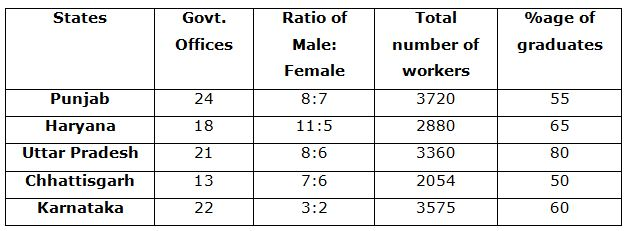# NIACL AO Prelims – Quantitative Aptitude Questions Day- 70

Dear Readers, Bank Exam Race for the Year 2019 is already started, To enrich your preparation here we have providing new series of Practice Questions on Quantitative Aptitude – Section. Candidates those who are preparing for NIACL AO Prelims 2019 Exams can practice these questions daily and make your preparation effective.

[WpProQuiz 4793]

Directions (1-5): Study the table carefully and answer the following questions.1) The number of male graduates in Uttar Pradesh is 1800. If the number of female graduate increase by 50% in the next year, approximately what percent of female workers in that particular state is graduate?

a) 93%

b) 95%

c) 87%

d) 125%

e) 75%

2) What is the ratio between the total number of male workers in states Haryana and Karnataka to the total number of graduate workers in the same states?

a) 1:2

b) 1375:1339

c) 2:5

d) 841:902

e) None of these

3) What is the total number of graduate workers from all the states?

a) 9778

b) 9017

c) 9885

d) 9025

e) None of these

4) Which state has the lowest number of average workers per office?

b) Karnataka

c) Haryana

d) Punjab

e) None of these

5) In which state percentage of female workers to number of workers (both male and female) is ranked third highest?

a) Chhattisgarh

b) Haryana

c) Punjab

e) None of these

Directions (6 -10): In the following questions, two equations I and II are given. You have to solve both the equations and give Answer as,

a) If x > y

b) If x ≥ y

c) If x < y

d) If x ≤ y

e) If x = y or the relation cannot be established

6)

I) x2 – 18x – 88 = 0

II) y2 – 13y – 68 = 0

7)

I) 3x – 5y = 8

II) 2x – 3y = 3

8)

I) 2x2 – 20x – 42 = 0

II) 3y2 – 29y + 56 = 0

9)

I) 5x2 – 33x + 52 = 0

II) 4y2 – 21y + 27 = 0

10)

I) 10x2  – 17x + 7 = 0

II) 15y2 – 19y + 6 = 0

Directions (1-5):

= (3360*80)/100 = 2688

Number of female graduates = 2688 – 1800 = 888

Females increases by 50% = (888*150)/100 = 1332

Total number of female in Uttar Pradesh = (6*3360)/14 = 1440

Required % = (1332*100)/1440 = 92.5% = 93% (approx.)

Required ratio

= [(11/16)*2880 + (3/5)*3575]/[(65/100)*2880 + (60/100)*3575]

= (1980+2145)/(1872+2145) = 4125/4017

= 1375:1339

Total number of graduate workers from all the states

= 3720*(55/100) + 2880*(65/100) + 3360*(80/100) + 2054*(50/100) + 3575*(60/100)

= (2046+1872+2688+1027+2145) = 9778

Punjab = 3720//24 = 155

Haryana = 2880/18 = 160

Uttar Pradesh = 3360/21 = 160

Chhattisgarh = 2054/13 = 158

Karnataka = 3575/22 =162

Hence, Punjab has the lowest number of average workers.

Punjab = 7/15*100 = 46.67%

Haryana = 5/16*100 = 31.25%

Uttar Pradesh = 6/14*100 = 42.86%

Chhattisgarh = 6/13*100 = 46.15%

Karnataka = 2/5*100 = 40%

Directions (6-10):

I) x2 – 18x – 88 = 0

(x – 22) (x + 4) = 0

X = 22, -4

II) y2 – 13y – 68 = 0

(y – 17) (y + 4) = 0

Y = 17, -4

Can’t be determined

3x – 5y = 8 –> (1)

2x – 3y = 3 –> (2)

By solving the equation (1) and (2), we get,

X = -9, y = -7

X < y

I) 2x2 – 20x – 42 = 0

2x2 – 14x – 6x – 42 = 0

2x (x – 7) – 6 (x – 7) = 0

(2x – 6) (x – 7) = 0

X = 6/2, 7 = 3, 7

II) 3y2 – 29y + 56 = 0

3y2 – 21y – 8y + 56 = 0

3y (y – 7) – 8 (y – 7) = 0

(3y – 8) (y – 7) = 0

Y = 8/3, 7 = 2.66, 7

Can’t be determined

I) 5x2 – 33x + 52 = 0

5x2 – 20x – 13x + 52 = 0

5x(x – 4) – 13 (x – 4) = 0

(5x – 13) (x – 4) = 0

X = 13/5, 4 = 2.6, 4

II) 4y2 – 21y + 27 = 0

4y2 – 12y – 9y + 27 = 0

4y (y – 3) – 9 (y – 3) = 0

(4y – 9) (y – 3) = 0

Y = 9/4, 3 = 2.25, 3

Can’t be determined

I) 10x2 – 17x + 7 = 0

10x2 – 10x – 7x + 7 = 0

10x(x – 1) – 7(x – 1) = 0

(10x – 7) (x – 1) = 0

X= 7/10, 1 = 0.7, 1

II) 15y2 – 19y + 6 = 0

15y2 -10y – 9y + 6 = 0

5y (3y – 2) – 3 (3y – 2) = 0

(5y – 3) (3y – 2) = 0

Y= 3/5, 2/3 = 0.6, 0.67

X > y